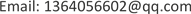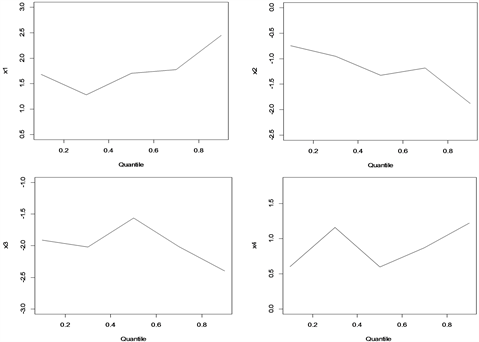﻿ FDI与金融发展对经济增长的影响研究 Research on the Influence of FDI and Financial Development on Economic Growth

Vol. 08  No. 07 ( 2019 ), Article ID: 31262 , 8 pages
10.12677/ASS.2019.87159

Research on the Influence of FDI and Financial Development on Economic Growth

Ling Xiao

Institute of Statistics, Jiangxi University of Finance and Economics, Nanchang JiangxiReceived: Jun. 21st, 2019; accepted: Jul. 5th, 2019; published: Jul. 15th, 2019ABSTRACT

The article uses the method of quantile regression and path analysis to study the complex relationship between FDI, financial development and economic growth. From the results of quantile regression, financial development and investment levels will hinder economic growth, and this hindrance is weakening; FDI and import and export will promote economic development, and this kind of promotion is increasing. From the research results of the path analysis method, FDI will promote the growth of China’s economy, and the indirect effect of FDI on economic growth is greater than the direct impact effect; financial development hinders the growth of China’s economy to a certain extent, and financial development for economic growth. The direct impact effect is greater than the indirect impact effect, and financial development can only promote economic growth if it reaches a certain threshold.

Keywords:FDI, Financial Development, Economic Growth, Quantile Regression, Path Analysis

FDI与金融发展对经济增长的影响研究1. 引言

2. 文献综述

2.1. 国外研究综述

2.2. 国内研究综述

3. 研究方法

3.1. 分位数回归

Roger Koenker和Gilbert Bassett在1978年提出了分位数回归的方法，与传统的回归分析不同的是，分位数回归是研究自变量与因变量之间的条件分位数之间的关系，从而得到由自变量估计因变量的条件分位数的回归模型。分位数回归不仅能得到因变量的中间趋势，还可以进一步推出因变量的条件分布概率。

$F\left(x\right)=P\left(X\le x\right)$ (1)

${Q}_{\tau }\left(X\right)=\mathrm{arg}\mathrm{inf}\left\{x\in R;F\left(x\right)\ge \tau \right\}\left(0<\tau <1\right)$ (2)

${F}_{X}^{-1}\left(\tau \right)=\mathrm{inf}\left\{y\in R;F\left(y\right)\ge \tau \right\}$ (3)

${Q}_{\tau }\left(X\right)={F}_{X}^{-1}\left(\tau \right)$ (4)

${\mathrm{min}}_{\beta \in {R}^{p}}\left[\underset{i\in \left\{i:{x}_{i}\ge \xi \right\}}{\sum }\tau |{x}_{i}-\xi |+\underset{i\in \left\{i:{x}_{i}<\xi \right\}}{\sum }\left(1-\tau \right)|{x}_{i}-\xi |\right]$ (5)

$\underset{\xi \in R}{\mathrm{min}}\underset{i=1}{\sum }{\rho }_{\tau }\left({x}_{i}-\xi \right)$ (6)

3.2. 通径分析

1) 首先要计算出各个变量间的相关系数，

2) 根据一个因变量和多个自变量，建立以下多元回归方程：

$\left\{\begin{array}{c}{P}_{1y}+{r}_{12}{P}_{2y}+\cdots +{r}_{1n}{P}_{ny}={r}_{1y}\\ {r}_{21}{P}_{1y}+{P}_{2y}+\cdots +{r}_{2n}{P}_{ny}={r}_{2y}\text{}\\ ⋮\\ {r}_{n1}{P}_{1y}+{r}_{n2}{P}_{2y}+\cdots +{P}_{ny}={r}_{ny}\text{}\end{array}$ (7)

3) 根据方程 ${r}_{iy}={P}_{yi}+\sum {P}_{j}{r}_{ij}$ ，计算各个自变量对因变量的直接通径系数和间接通径系数，最后得出

4. FDI与金融业对经济增长的实证分析

4.1. 指标选取

4.2. 分位数回归Figure 1. Quantile regression coefficient fluctuation chart

5. 经济增长影响因素的通径分析

5.1. 最小二乘法回归

$y=1.12×{10}^{-15}+1.601{x}_{1}-1.128{x}_{2}-1.93{x}_{3}+0.9552{x}_{4}$ (8)

5.2. 通径分析

6. 结论与建议

6.1. 结论

1) 分位数回归结果

2) 通径分析结果

FDI为我国的经济增长提供了有利的条件，且FDI对经济增长的间接影响效应大于直接影响效应。FDI对经济增长的间接影响效应中，金融发展(社会融资规模)和投资水平(固定资产投资额)两者的作用较大，而通过进出口额的影响效应较小。

6.2. 建议

Research on the Influence of FDI and Financial Development on Economic Growth[J]. 社会科学前沿, 2019, 08(07): 1149-1156. https://doi.org/10.12677/ASS.2019.87159

1. 1. Aghion, P., Howit, P. and Mayer-Foulkers, D. (2005) The Effect of Financial Development on Convergence: Theory and Evidence. Quarterly Journal of Economics, 120, 173-222. https://doi.org/10.1162/qjec.2005.120.1.173

2. 2. Roussean, P.L. and Wachtel, P. (2011) What Is Happening to the Impact of Financial Deepening on Economic Growth? Economic Inquiry, 49, 276-288. https://doi.org/10.1111/j.1465-7295.2009.00197.x

3. 3. Blomstrom, M. and Kokko, A. (1998) Multinational Corporations and Spillovers. Journal of Economic Surveys, 12, 247-277. https://doi.org/10.1111/1467-6419.00056

4. 4. Alfaro, L., Kalemli-Ozcan, S. and Sayek, S. (2009) FDI, Productivity and Financial Development. The World Economy, 32, 111-135. https://doi.org/10.1111/j.1467-9701.2009.01159.x

5. 5. 徐临, 王明利, 秦莎. 关于京津冀地区FDI、金融发展与经济增长的关系研究[J]. 金融教育研究, 2018, 31(1): 38-44.

6. 6. 王芳, 刘伟宏. 区域金融发展和经济增长的计量分析[J]. 海峡科学, 2013, 3(75): 8-10.

7. 7. 陈伟, 徐剑, 唐含宇. 发展中国家的金融发展、FDI与经济增长[J]. 现代管理科学, 2014(7): 43-45.

8. 8. 金山, 汪前元. FDI、金融发展与经济增长——一个分析框架及基于中国数据的经验检验[J]. 开放导报, 2012(1): 80-84.